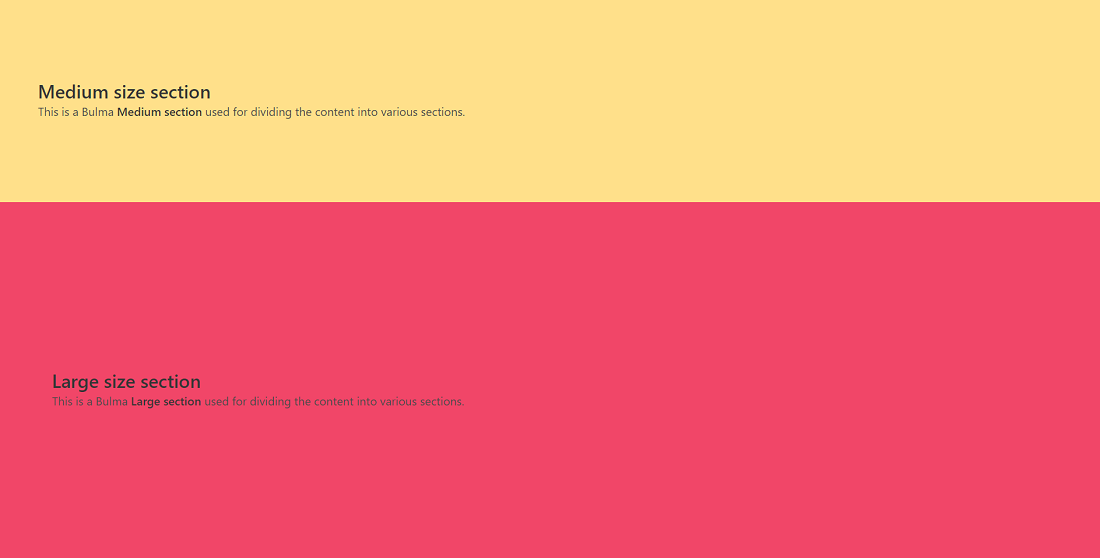GFG App
Open AppBrowser
Continue

In this article, we’ll be seeing the Bulma section. A Section is a basic container that is used to divide the webpage content into different sections.

Bulma Section class:

• section- This class of Bulma is used to divide the webpage into different sections and has less padding.
• section is-medium- This class of Bulma is used to divide the webpage into different sections in a medium size.
• section is-large- This class of Bulma is used to divide the webpage into different sections in a large size.

Syntax:

```<section class="section ||
section is-medium ||
section is-large">
......
</section>```

Example 1: Below example illustrates the section in Bulma.

## HTML

 ` ` `<``html` `lang``=``"en"``> ` ` `  `<``head``> ` `  ``<``link` `rel``=``"stylesheet"` `href``= ` `"https://cdn.jsdelivr.net/npm/bulma@0.9.3/css/bulma.min.css"``> ` ` ` ` `  `<``body``> ` `  ``<``section` `class``=``"section has-background-primary"``> ` `    ``<``h1` `class``=``"title"``> ` `      ``GeeksforGeeks ` `    `` ` ` `  `    ``<``h2` `class``=``"subtitle"``> ` `      ``This is a Bulma  ` `      ``<``strong``>section  ` `      ``used for dividing the content  ` `      ``into various sections. ` `    `` ` `  `` ` ` ` ` `  ``

Output:Example 2: Below example illustrates the two sizes of the section class in Bulma.

## HTML

 ` ` `<``html` `lang``=``"en"``> ` ` `  `  ``<``head``> ` `    ``<``link` `rel``=``"stylesheet"` `href``= ` `"https://cdn.jsdelivr.net/npm/bulma@0.9.3/css/bulma.min.css"``/> ` `  `` ` ` `  `  ``<``body``> ` `    ``<``section` `class="section is-medium  ` `      ``has-background-warning"> ` `      ``<``h1` `class``=``"title"``> ` `        ``Medium size section ` `      `` ` ` `  `      ``<``h2` `class``=``"subtitle"``> ` `        ``This is a Bulma  ` `        ``<``strong``>Medium section  ` `        ``used for dividing the content ` `        ``into various sections. ` `      `` ` `    `` ` ` `  `    ``<``section` `class="section is-large  ` `      ``has-background-danger"> ` `      ``<``h1` `class``=``"title"``>Large size section ` `      ``<``h2` `class``=``"subtitle"``> ` `        ``This is a Bulma  ` `        ``<``strong``>Large section  ` `        ``used for dividing the ` `        ``content into various sections. ` `      `` ` `    `` ` `  `` ` `   `  ``

Output:My Personal Notes arrow_drop_up